# Image Approximation with Fourier and Wavelets¶

Important: Please read the installation page for details about how to install the toolboxes. $\newcommand{\dotp}{\langle #1, #2 \rangle}$ $\newcommand{\enscond}{\lbrace #1, #2 \rbrace}$ $\newcommand{\pd}{ \frac{ \partial #1}{\partial #2} }$ $\newcommand{\umin}{\underset{#1}{\min}\;}$ $\newcommand{\umax}{\underset{#1}{\max}\;}$ $\newcommand{\umin}{\underset{#1}{\min}\;}$ $\newcommand{\uargmin}{\underset{#1}{argmin}\;}$ $\newcommand{\norm}{\|#1\|}$ $\newcommand{\abs}{\left|#1\right|}$ $\newcommand{\choice}{ \left\{ \begin{array}{l} #1 \end{array} \right. }$ $\newcommand{\pa}{\left(#1\right)}$ $\newcommand{\diag}{{diag}\left( #1 \right)}$ $\newcommand{\qandq}{\quad\text{and}\quad}$ $\newcommand{\qwhereq}{\quad\text{where}\quad}$ $\newcommand{\qifq}{ \quad \text{if} \quad }$ $\newcommand{\qarrq}{ \quad \Longrightarrow \quad }$ $\newcommand{\ZZ}{\mathbb{Z}}$ $\newcommand{\CC}{\mathbb{C}}$ $\newcommand{\RR}{\mathbb{R}}$ $\newcommand{\EE}{\mathbb{E}}$ $\newcommand{\Zz}{\mathcal{Z}}$ $\newcommand{\Ww}{\mathcal{W}}$ $\newcommand{\Vv}{\mathcal{V}}$ $\newcommand{\Nn}{\mathcal{N}}$ $\newcommand{\NN}{\mathcal{N}}$ $\newcommand{\Hh}{\mathcal{H}}$ $\newcommand{\Bb}{\mathcal{B}}$ $\newcommand{\Ee}{\mathcal{E}}$ $\newcommand{\Cc}{\mathcal{C}}$ $\newcommand{\Gg}{\mathcal{G}}$ $\newcommand{\Ss}{\mathcal{S}}$ $\newcommand{\Pp}{\mathcal{P}}$ $\newcommand{\Ff}{\mathcal{F}}$ $\newcommand{\Xx}{\mathcal{X}}$ $\newcommand{\Mm}{\mathcal{M}}$ $\newcommand{\Ii}{\mathcal{I}}$ $\newcommand{\Dd}{\mathcal{D}}$ $\newcommand{\Ll}{\mathcal{L}}$ $\newcommand{\Tt}{\mathcal{T}}$ $\newcommand{\si}{\sigma}$ $\newcommand{\al}{\alpha}$ $\newcommand{\la}{\lambda}$ $\newcommand{\ga}{\gamma}$ $\newcommand{\Ga}{\Gamma}$ $\newcommand{\La}{\Lambda}$ $\newcommand{\si}{\sigma}$ $\newcommand{\Si}{\Sigma}$ $\newcommand{\be}{\beta}$ $\newcommand{\de}{\delta}$ $\newcommand{\De}{\Delta}$ $\newcommand{\phi}{\varphi}$ $\newcommand{\th}{\theta}$ $\newcommand{\om}{\omega}$ $\newcommand{\Om}{\Omega}$

This numerical tour overviews the use of Fourier and wavelets for image approximation.

In :
library(pracma)
library(imager)

# Importing the libraries
for (f in list.files(path="nt_toolbox/toolbox_general/", pattern="*.R")) {
source(paste("nt_toolbox/toolbox_general/", f, sep=""))
}
for (f in list.files(path="nt_toolbox/toolbox_signal/", pattern="*.R")) {
source(paste("nt_toolbox/toolbox_signal/", f, sep=""))
}

Loading required package: plyr

Attaching package: ‘magrittr’

The following objects are masked from ‘package:pracma’:

and, mod, or

Attaching package: ‘imager’

The following object is masked from ‘package:magrittr’:

The following object is masked from ‘package:plyr’:

liply

The following objects are masked from ‘package:stats’:

convolve, spectrum

The following object is masked from ‘package:graphics’:

frame

The following object is masked from ‘package:base’:

save.image

Attaching package: ‘tuneR’

The following objects are masked from ‘package:imager’:

channel, play

Attaching package: ‘akima’

The following object is masked from ‘package:imager’:

interp



Note: to measure the error of an image $f$ with its approximation $f_M$, we use the SNR measure, defined as

$$\text{SNR}(f,f_M) = -20\log_{10} \pa{ \frac{ \norm{f-f_M} }{ \norm{f} } },$$

which is a quantity expressed in decibels (dB). The higer the SNR, the better the quality.

First we load an image $f \in \RR^N$ of $N = N_0 \times N_0$ pixels.

In :
n = 512


Display the original image.

In :
options(repr.plot.width=4, repr.plot.height=4)
imageplot(f, 'Image f')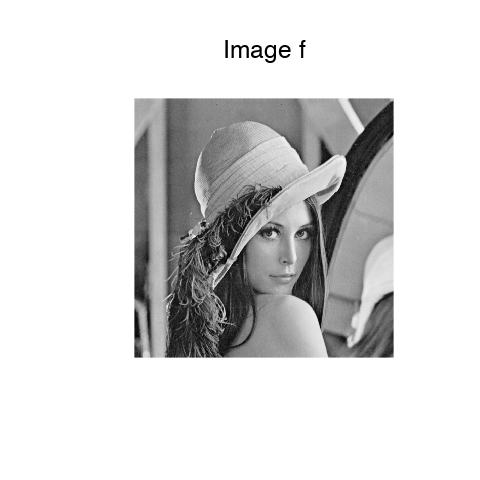Display a zoom in the middle.

In :
imageplot(f[c(((n/2) - 32):((n/2) + 32)), c(((n/2) - 32):((n/2) + 32))], 'Zoom')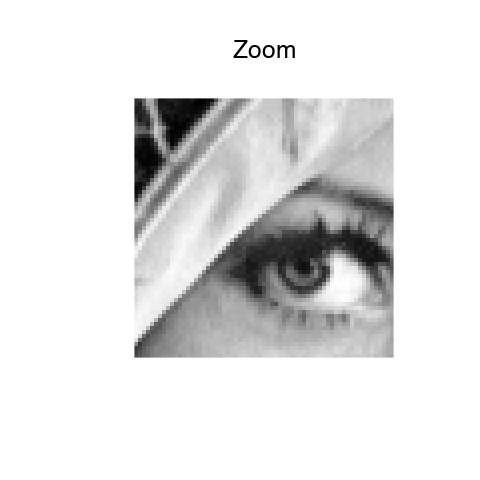An image is a 2D array, it can be modified as a matrix.

In :
options(repr.plot.width=5, repr.plot.height=5)
imageplot(-f, '-f', c(1, 2, 1))
imageplot(f[dim(f):1,], 'Flipped', c(1, 2, 2))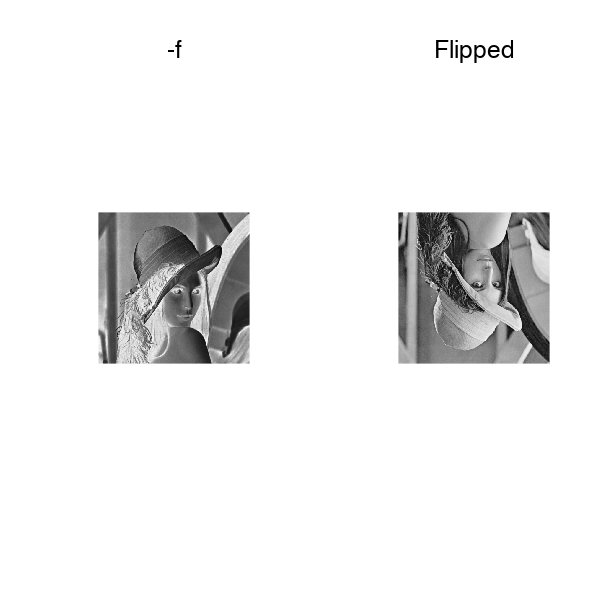Blurring is achieved by computing a convolution $f \star h$ with a kernel $h$.

Compute the low pass kernel.

In :
k = 9
h = matrix(1, k, k)
h = h / sum(h) #normalize


Compute the convolution $f \star h$.

In :
fh = convolution(f[,], h)


Display.

In :
imageplot(fh, 'Blurred image')## Fourier Transform¶

The Fourier orthonormal basis is defined as $$\psi_m(k) = \frac{1}{\sqrt{N}}e^{\frac{2i\pi}{N_0} \dotp{m}{k} }$$ where $0 \leq k_1,k_2 < N_0$ are position indexes, and $0 \leq m_1,m_2 < N_0$ are frequency indexes.

The Fourier transform $\hat f$ is the projection of the image on this Fourier basis

$$\hat f(m) = \dotp{f}{\psi_m}.$$

The Fourier transform is computed in $O(N \log(N))$ operation using the FFT algorithm (Fast Fourier Transform). Note the normalization by $\sqrt{N}=N_0$ to make the transform orthonormal.

In :
F = fft(f[,]) / n


We check this conservation of the energy.

In :
print(paste("Energy of Image: ", toString(norm(f[,]))))
print(paste("Energy of Fourier: ", toString(norm(Mod(F[,])))))

 "Energy of Image:  279.584762635359"
 "Energy of Fourier:  279.584762635359"


Compute the logarithm of the Fourier magnitude $\log\left(\abs{\hat f(m)} + \epsilon\right)$, for some small $\epsilon$.

In :
L = fftshift(log(Mod(F) + 1e-1))


Display. Note that we use the function fftshift to put the 0 low frequency in the middle.

In :
imageplot(L, 'Log(Fourier transform)')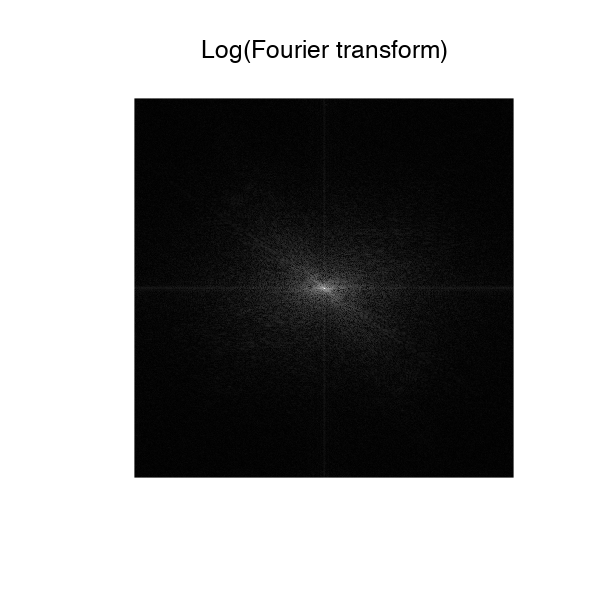## Linear Fourier Approximation¶

An approximation is obtained by retaining a certain set of index $I_M$

$$f_M = \sum_{ m \in I_M } \dotp{f}{\psi_m} \psi_m.$$

Linear approximation is obtained by retaining a fixed set $I_M$ of $M = \abs{I_M}$ coefficients. The important point is that $I_M$ does not depend on the image $f$ to be approximated.

For the Fourier transform, a low pass linear approximation is obtained by keeping only the frequencies within a square.

$$I_M = \enscond{m=(m_1,m_2)}{ -q/2 \leq m_1,m_2 < q/2 }$$

where $q = \sqrt{M}$.

This can be achieved by computing the Fourier transform, setting to zero the $N-M$ coefficients outside the square $I_M$ and then inverting the Fourier transform.

Number $M$ of kept coefficients.

In :
M = n**2 / 64


Exercise 1

Perform the linear Fourier approximation with $M$ coefficients. Store the result in the variable $f_M$.

In :
source("nt_solutions/introduction_4_fourier_wavelets/exo1.R")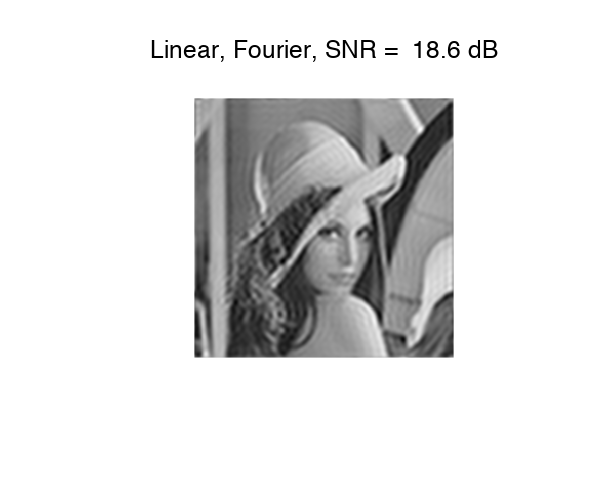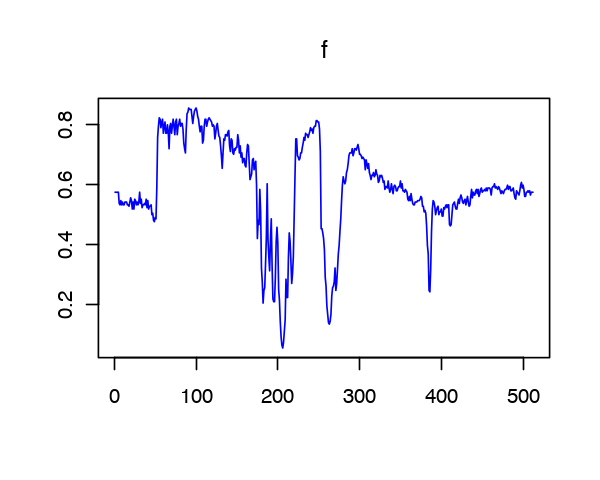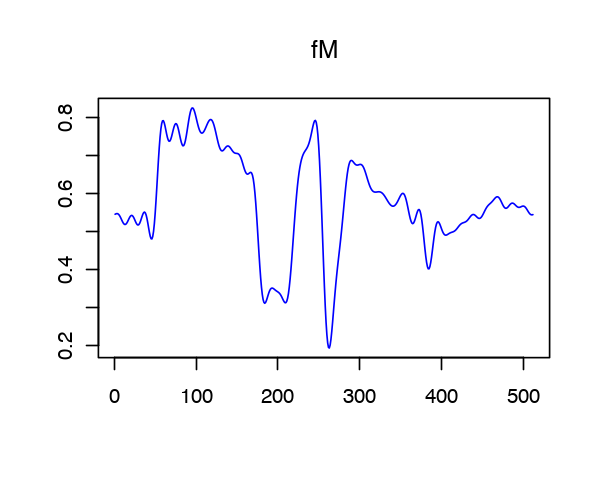In :
# Insert your code here.


Compare two 1D profile (lines of the image). This shows the strong ringing artifact of the linea approximation.

In :
# Insert your code here.


## Non-linear Fourier Approximation¶

Non-linear approximation is obtained by keeping the $M$ largest coefficients. This is equivalently computed using a thresholding of the coefficients $$I_M = \enscond{m}{ \abs{\dotp{f}{\psi_m}}>T }.$$

Set a threshold $T>0$.

In :
T = 0.2


Compute the Fourier transform.

In :
F = fft(f) / n


Do the hard thresholding.

In :
FT = F * (abs(F) > T)


Display. Note that we use the function fftshift to put the 0 low frequency in the middle.

In :
L = fftshift(log(abs(FT[,]) + 1e-1))
imageplot(L, 'thresholded Log(Fourier transform)')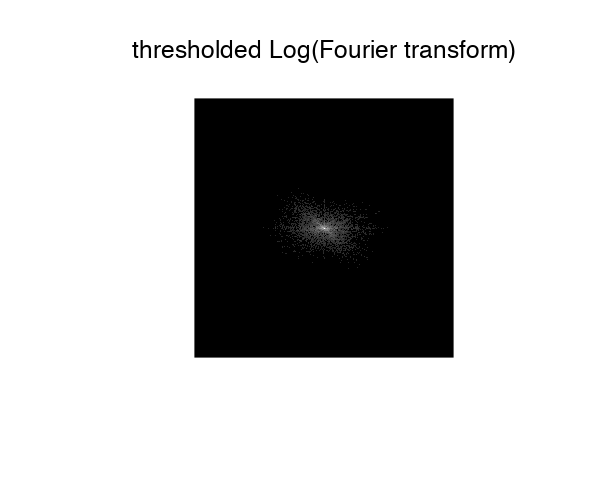Inverse Fourier transform to obtain $f_M$.

In :
fM = Re(fft(FT, inverse=TRUE) * n)
# Normalize to 0-1
fM = (fM-min(fM))/(max(fM)-min(fM))


Display.

In :
imageplot(fM, paste("Non - Linear, Fourier, SNR = ",  round(snr(f, fM), 1), "dB"))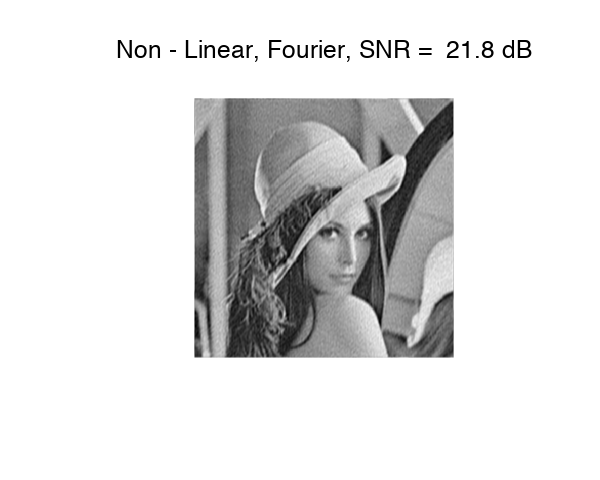Given a $T$, the number of coefficients is obtained by counting the non-thresholded coefficients $\abs{I_M}$.

In :
m = sum(FT != 0)
print(paste("M/N = 1 /",as.integer(n**2/m)))

 "M/N = 1 / 30"


Exercise 2

Compute the value of the threshold $T$ so that the number of coefficients is $M$. Display the corresponding approximation $f_M$.

In :
source("nt_solutions/introduction_4_fourier_wavelets/exo2.R")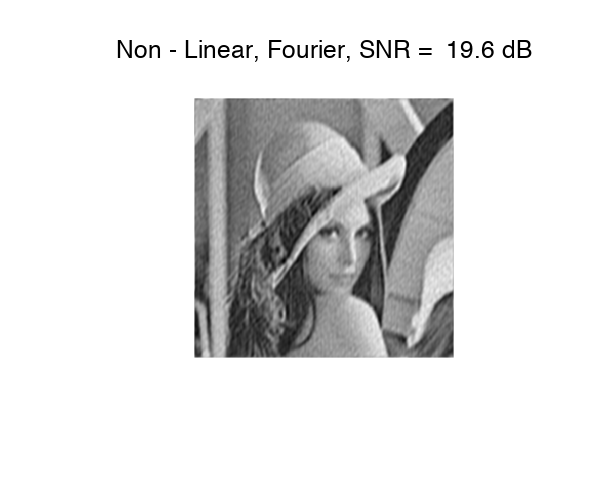In :
# Insert your code here.


## Wavelet Transform¶

A wavelet basis $\Bb = \{ \psi_m \}_m$ is obtained over the continuous domain by translating and dilating three mother wavelet functions $\{\psi^V,\psi^H,\psi^D\}$.

Each wavelet atom is defined as $$\psi_m(x) = \psi_{j,n}^k(x) = \frac{1}{2^j}\psi^k\pa{ \frac{x-2^j n}{2^j} }$$

The scale (size of the support) is $2^j$ and the position is $2^j(n_1,n_2)$. The index is $m=(k,j,n)$ for $\{ j \leq 0 \}$.

The wavelet transform computes all the inner products $\{ \dotp{f}{\psi_{j,n}^k} \}_{k,j,n}$.

Set the minimum scale for the transform to be 0.

In :
Jmin = 0


Perform the wavelet transform, $f_w$ stores all the wavelet coefficients.

In :
fw = perform_wavelet_transf(f, Jmin + 1, 1)


Display the transformed coefficients.

In :
options(repr.plot.width=6, repr.plot.height=6)
plot_wavelet(fw)
title(main="Wavelet coefficients")